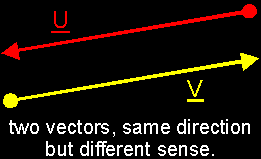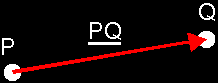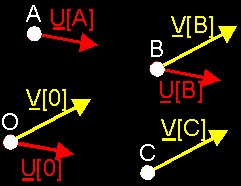3D Geometry Primer: Chapter 1 - Issue 01 - Introduction To Vectors by (14 August 2000) Return to The Archives
 IntroductionWhen we want to play around with geometry, we need a space to work in. Normally, for our purposes, this is 2D space or 3D space. It might sound a bit weird to talk about a 2D "space", while its still as flat as the plane. It's just so that for higher dimensions (4D, 5D, ..., nD) we also talk about 4D space, 5D space, ..., nD space; so it's logical to talk about 2D space too. And in that way, we don't confuse 2D space with a ordinary plane in 3D.Once you've got your space, you can make geometrical drawings and examine attributes of triangles, circles and other stuff. That might be enough for those math freaks (who I'm not :) to play around with their axioms, but we need something more. If we want to use geometry in the computer, we need a way to store information about it: where, what, when and why things are.In fact, there's only one type of element in our space: points. We write down points with capital letters: A, B, C, ..., P, ... There’s one "special" point in each space: the origin O .With points, we can make lines, planes, spheres, pyramids, just about anything. So, if we can store points, we can describe all known objects in our world. But how do we do it? We need to introduce vectors first. (Vectors will be capable of representing points, and with points we can make anything.)

 (I) What Is A Vector?Many tutorials describe a vector as a translation (an arrow) from one point to another and then they say it's a kind of script defining the translation you can apply on all other points. I'm not very happy with that description, because many potential usages of vectors get lost with such a narrow definition. I'll try something else...Basically there are 2 kinds of quantities. First, you have the scalar quantities such as temperature. You can say: "At this point, there's a temperature of 25°C" and that's it. You know everything about the temperature at that point. There's nothing more to say. With each point in the universe, you can couple one scalar. That's why they are called scalar quantities.Second, you have the vector quantities such as velocities. If you try to say: "At this point, a force pulls with a magnitude of 100 N (Newton)", then you don't know enough. In what direction is the point being pulled? You don't know anything about that. What we really want to say is: "At this point a force with a magnitude of 100 N pulls to the right".So, a vector can represent a quantity with a direction. It is like an (invisible) arrow: from a certain starting point, it points towards something with a certain length. That starting point is the point we talk about, the direction of the arrow is the direction of the quantity, and the length is its magnitude.To continue, I'm going to give a more proper definition of the vector we just saw. And actually, we have 2 kinds of vectors: the bound vectors and the free vectors. We'll start with the bound ones.

 (II) Bound VectorsA bound vector V has 4 attributes: 1. a starting point P: this is where expressed quantity (e.g. the force, the acceleration, the replacement) "attacks" 2. a direction: a vector lays always on top of 1 line. This line is the direction. (e.g. horizontal) 3. a sense: on that line, the vector can pull in 2 ways (e.g. to the left, to the right). The sense determines which one. 4. a magnitude: the length of the arrow (the magnitude of the force, of the acceleration). Remarks ° Parallel lines represent only 1 direction. So all vectors that have parallel directions have the same direction. ° Notice the difference between direction and sense!! The direction is a line, and the sense just determines the "good side of P on the line". e.g. If the direction is a horizontal line, then the sense can be to the right, or to the left.° Notice also that in principle a vector has no ending point! You need to see it this way: If you say "At this point the temperature is 25°C", you give information about only 1 point. With vectors you do the same thing. If you say "At this point, there's a force of 100 N that pulls to the right", you do not talk about the ending point. There might be a solid wall or even a vacüum at the ending point, but you’re not interested in that. The only thing you want to know is that a force attacks in the starting point with a certain magnitude and a certain direction. Therefore, we call the ending point a virtual point, because it doesn't need to have a real physical meaning.However, sometimes it is easier to talk about a vector, using its starting point and its (virtual) ending point. We also use the words tail (=starting point) and head (=ending point). From the moment you know the tail and head, you can easily find the direction, the sense, and the magnitude. Thus we can say about a vector: it points from its tail to its head. Nevertheless, we'll wait with that kind of storage, because its easier to explain vector arithmetic with the original definition. ° We write down a vector with an underlined capital: A, B, ..., V, ... If you explicit want to talk about the vector between its tail P and head Q, you can write: PQ° We write down the magnitude of a vector like ||V||. This is the "value" of the vector

 (III) Free VectorsSometimes a vector has no real starting point. If all points in your space move in the same direction, same sense and with the same speed; it would be a bit stupid to write down a velocity vector for each point. Then, we use free vectors. These are vectors with a direction, sense and magnitude but no starting point (tail). If you take all vectors with the same direction, sense and length but with a different starting point, and throw away all starting points, you get one and the same free vector. Thus, a free vector can be seen as a "set" of bound vectors with a given direction, sense and length, but with different starting points. We call each bound vector a representative of that set.To depict free vectors, one usually takes representatives with the same starting point, and one often calls that "special" starting point the origin O.If we want to distinguish the different representatives of a free vector, we give an index to each representative: the starting point. Sometimes, the free vector itself, we give the index 0 (zero). e.g. the representative of the free vector V with starting point A is V[A].(IV) Unit Vectors And Null VectorsUnit vector: Each vector with magnitude 1 is a unit vector. Those vectors will be very important in further geometry, because you can measure distances with it. Later, we’ll find a way to make a unit vector out of a arbitrary vector. We call this normalizing.Null vector: The null vector is a vector with magnitude 0, so the null vector is a point only. Notice that you can't define a direction nor a sense to the null vector. We write down the null vector as 0 (zero) or O (origin).Now, we have defined what a vector is, but we still can't store it. We need a way to translate vectors to values, because we can only store values in a computer. Thus, we need a numerical representation of a vector. And to find that, we first need vector arithmetic. (With vector arithmetic, we'll be able to represent vectors in our computer. With vectors we can represent points and with points we can represent anything. So we can represent anything with vector arithmetic :)But that's something for next week.Regards, Bramz